# Visualizing Time Series

This tutorial focuses on, `plot_time_series()`, a workhorse time-series plotting function that:

• Generates interactive `plotly` plots (great for exploring & shiny apps)
• Consolidates 20+ lines of `ggplot2` & `plotly` code
• Scales well to many time series
• Can be converted from interactive `plotly` to static `ggplot2` plots

## Libraries

Run the following code to setup for this tutorial.

``````library(dplyr)
library(ggplot2)
library(lubridate)
library(timetk)

# Setup for the plotly charts (# FALSE returns ggplots)
interactive <- FALSE``````

## Plotting Time Series

Let’s start with a popular time series, `taylor_30_min`, which includes energy demand in megawatts at a sampling interval of 30-minutes. This is a single time series.

``````taylor_30_min
#> # A tibble: 4,032 × 2
#>    date                value
#>    <dttm>              <dbl>
#>  1 2000-06-05 00:00:00 22262
#>  2 2000-06-05 00:30:00 21756
#>  3 2000-06-05 01:00:00 22247
#>  4 2000-06-05 01:30:00 22759
#>  5 2000-06-05 02:00:00 22549
#>  6 2000-06-05 02:30:00 22313
#>  7 2000-06-05 03:00:00 22128
#>  8 2000-06-05 03:30:00 21860
#>  9 2000-06-05 04:00:00 21751
#> 10 2000-06-05 04:30:00 21336
#> # ℹ 4,022 more rows``````

The `plot_time_series()` function generates an interactive `plotly` chart by default.

• Simply provide the date variable (time-based column, `.date_var`) and the numeric variable (`.value`) that changes over time as the first 2 arguments
• When `.interactive = TRUE`, the `.plotly_slider = TRUE` adds a date slider to the bottom of the chart.
``````taylor_30_min %>%
plot_time_series(date, value,
.interactive = interactive,
.plotly_slider = TRUE)``````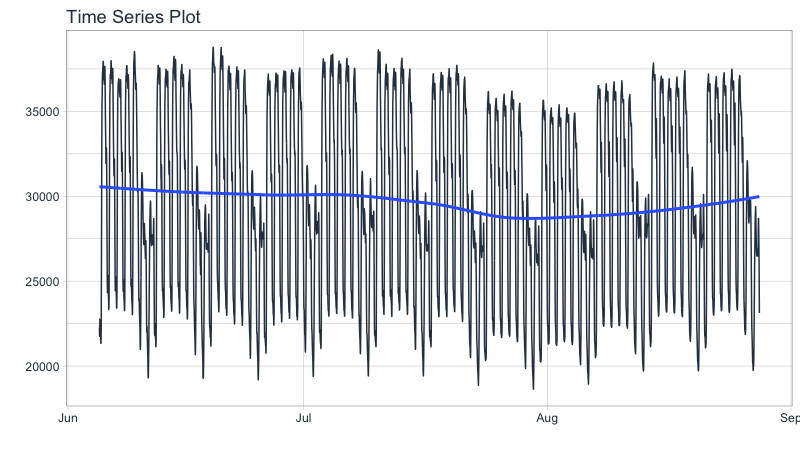### Plotting Groups

Next, let’s move on to a dataset with time series groups, `m4_daily`, which is a sample of 4 time series from the M4 competition that are sampled at a daily frequency.

``````m4_daily %>% group_by(id)
#> # A tibble: 9,743 × 3
#> # Groups:   id 
#>    id    date       value
#>    <fct> <date>     <dbl>
#>  1 D10   2014-07-03 2076.
#>  2 D10   2014-07-04 2073.
#>  3 D10   2014-07-05 2049.
#>  4 D10   2014-07-06 2049.
#>  5 D10   2014-07-07 2006.
#>  6 D10   2014-07-08 2018.
#>  7 D10   2014-07-09 2019.
#>  8 D10   2014-07-10 2007.
#>  9 D10   2014-07-11 2010
#> 10 D10   2014-07-12 2002.
#> # ℹ 9,733 more rows``````

Visualizing grouped data is as simple as grouping the data set with `group_by()` prior to piping into the `plot_time_series()` function. Key points:

• Groups can be added in 2 ways: by `group_by()` or by using the `...` to add groups.
• Groups are then converted to facets.
• `.facet_ncol = 2` returns a 2-column faceted plot
• `.facet_scales = "free"` allows the x and y-axis of each plot to scale independently of the other plots
``````m4_daily %>%
group_by(id) %>%
plot_time_series(date, value,
.facet_ncol = 2, .facet_scales = "free",
.interactive = interactive)``````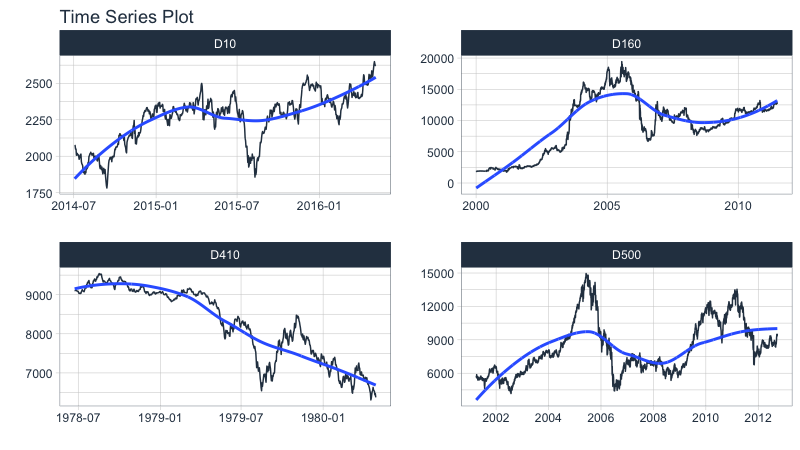### Visualizing Transformations & Sub-Groups

Let’s switch to an hourly dataset with multiple groups. We can showcase:

1. Log transformation to the `.value`
2. Use of `.color_var` to highlight sub-groups.
``````m4_hourly %>% group_by(id)
#> # A tibble: 3,060 × 3
#> # Groups:   id 
#>    id    date                value
#>    <fct> <dttm>              <dbl>
#>  1 H10   2015-07-01 12:00:00   513
#>  2 H10   2015-07-01 13:00:00   512
#>  3 H10   2015-07-01 14:00:00   506
#>  4 H10   2015-07-01 15:00:00   500
#>  5 H10   2015-07-01 16:00:00   490
#>  6 H10   2015-07-01 17:00:00   484
#>  7 H10   2015-07-01 18:00:00   467
#>  8 H10   2015-07-01 19:00:00   446
#>  9 H10   2015-07-01 20:00:00   434
#> 10 H10   2015-07-01 21:00:00   422
#> # ℹ 3,050 more rows``````

The intent is to showcase the groups in faceted plots, but to highlight weekly windows (sub-groups) within the data while simultaneously doing a `log()` transformation to the value. This is simple to do:

1. `.value = log(value)` Applies the Log Transformation
2. `.color_var = week(date)` The date column is transformed to a `lubridate::week()` number. The color is applied to each of the week numbers.
``````m4_hourly %>%
group_by(id) %>%
plot_time_series(date, log(value),             # Apply a Log Transformation
.color_var = week(date),      # Color applied to Week transformation
# Facet formatting
.facet_ncol = 2,
.facet_scales = "free",
.interactive = interactive)``````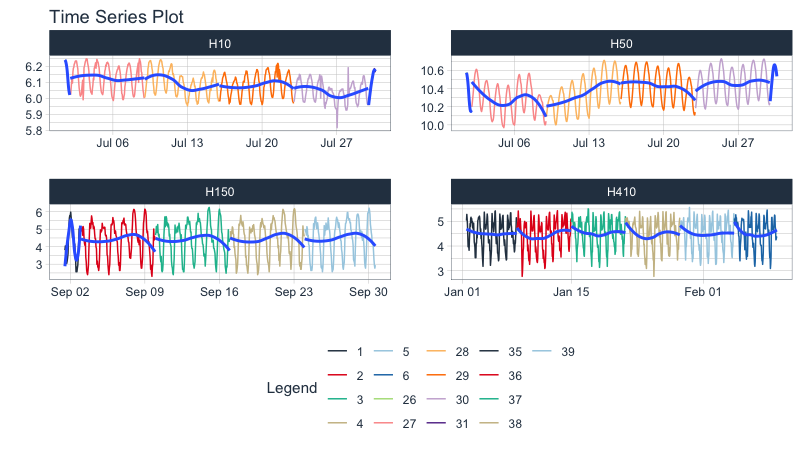### Static ggplot2 Visualizations & Customizations

All of the visualizations can be converted from interactive `plotly` (great for exploring and shiny apps) to static `ggplot2` visualizations (great for reports).

``````taylor_30_min %>%
plot_time_series(date, value,
.color_var = month(date, label = TRUE),

# Returns static ggplot
.interactive = FALSE,

# Customization
.title = "Taylor's MegaWatt Data",
.x_lab = "Date (30-min intervals)",
.y_lab = "Energy Demand (MW)",
.color_lab = "Month") +
scale_y_continuous(labels = scales::comma_format())``````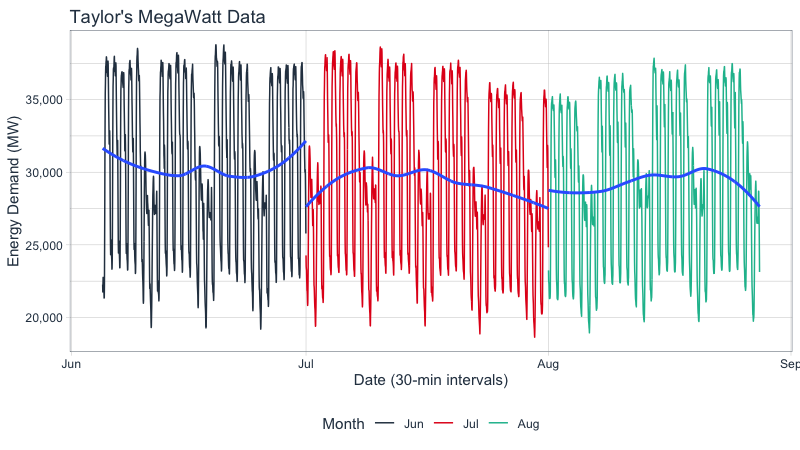## Box Plots (Time Series)

The `plot_time_series_boxplot()` function can be used to make box plots.

• Box plots use an aggregation, which is a key parameter defined by the `.period` argument.
``````m4_monthly %>%
group_by(id) %>%
plot_time_series_boxplot(
date, value,
.period      = "1 year",
.facet_ncol  = 2,
.interactive = FALSE)``````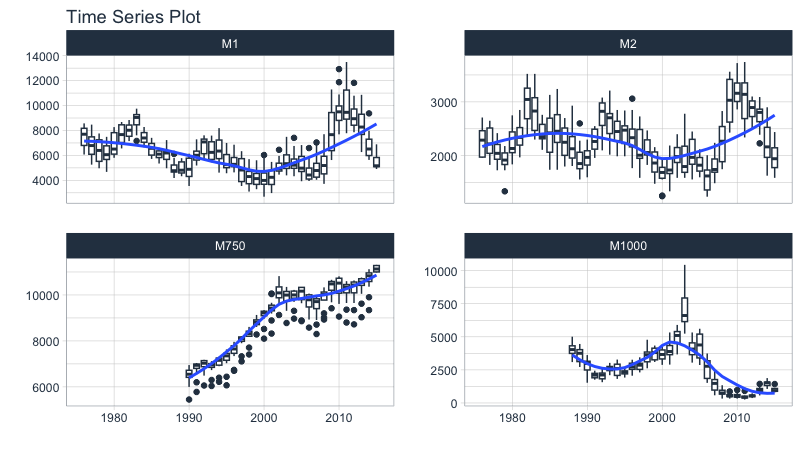## Regression Plots (Time Series)

A time series regression plot, `plot_time_series_regression()`, can be useful to quickly assess key features that are correlated to a time series.

• Internally the function passes a `formula` to the `stats::lm()` function.
• A linear regression summary can be output by toggling `show_summary = TRUE`.
``````m4_monthly %>%
group_by(id) %>%
plot_time_series_regression(
.date_var     = date,
.formula      = log(value) ~ as.numeric(date) + month(date, label = TRUE),
.facet_ncol   = 2,
.interactive  = FALSE,
.show_summary = FALSE
)``````